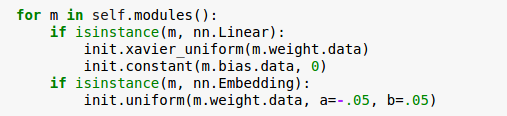# My first time using torch and i want to know is there anything wrong in my implementation?

Actually i am a very beginner in torchi made the model in keras and it went well but in pytorch it is not converging
i just want to know is it an error or something that i don’t know about torch
the input is the question and the output is answer.

``````class Classifier(nn.Module):

def __init__(self, num_labels= 503, vocab_size= 880):

super(Classifier, self).__init__()

self.embed = nn.Embedding(vocab_size, 128)
self.linear1 = nn.Linear(128, 128)
self.linear2 = nn.Linear(128, num_labels)

def forward(self, bow_vec):
layer1 = self.embed(bow_vec)
layer2 = layer1.sum(1).squeeze(1)

layer3 = F.relu(self.linear1(layer2))
layer3 = F.relu(self.linear1(layer3))
layer4 = self.linear2(layer3)
return layer4

losses = []
loss_function = nn.CrossEntropyLoss()
model = Classifier()
for epoch in range(50):
total_loss = torch.Tensor()

inputs, labels = Variable(x), Variable(y, requires_grad= False)

log_probs = model(inputs)

loss = loss_function(log_probs, labels)

# Step 5. Do the backward pass and update the gradient
loss.backward()
optimizer.step()

total_loss += loss.data
losses.append(total_loss)
print(losses)
``````

btw new_y is a vector of the target indices.
sorry for that long code but this is my first time
thanksAre you sure `x` and `y` are a one-to-one match?
Is learning rate the same as you do in keras?
`layer3 = F.relu(self.linear1(layer3))` are you sure to use `self.linear1` twice?
you can also add print in `forward` to make sure the number does not overflow

``````    def forward(self, bow_vec):
layer1 = self.embed(bow_vec)
layer2 = layer1.sum(1).squeeze(1)
print layer2.data #.....
layer3 = F.relu(self.linear1(layer2))
layer3 = F.relu(self.linear1(layer3))
layer4 = self.linear2(layer3)
return layer4
``````
1 Like

First thank you so much
but what is the meaning of “x and y are a one-to-one match” ?

if x is data and y is label, usually we would put them in one dataset to make sure that y is the label of x, especially when you want to use shuffle sometimes. But I guess it’s ok here.
Also try init the linear layers with methods from torch.nn.init

1 Like

i made sure my code is exactly the same as keras code,
THE DIFFERENCE NOW IS that the model now in keras just give better results after the same number of epochs,
ex:
after 15 epochs the accuracy is:
keras code gave 27.5%
pytorch code gave 22.5%

it would be really great if you have any advice for medid you take this advice from chenyuntc ?

Also try init the linear layers with methods from torch.nn.init

there is probably still difference in your model.

1 Like

yesIt’s hard to say, but there are so many details to look at carefully.
Such as optimization methods, do you use weight_decay(default 0 in PyTorch), is `betas` the same in Adam.
Besides, do you use the same validate dataset, do you have the same batch size(the same epoch, too large batch_size usually would converge slower)?.
The initialization of `embedding` layer also seems too small for me, is this the same as you do in keras?

Generall, it won’t matter so much if the converge rates are close since PyTorch is much faster than keras1 Like